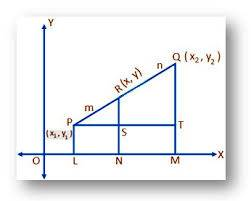# What does negative ratio mean in mathematics?

• I
Whats the difference between (4/3) and -(4/3)

Why ratio in external division is negative?

I have answer for how it is negative but not why?
X = (mx2+nx1)/(m+n)

⇒ (mx2+nx1) = X(m+n)
⇒m(x2-X) = n(X-x1)
⇒(m/n) = (X-x1)/(x2-X)

From the above equation we can conclude that when division is external then X must be greater than x1 and x2, therefore ratio becomes negative
But whats the purpose of negative ratio.

hilbert2
Gold Member
A ratio is negative when the divisor and dividend are of different sign.

A ratio is negative when the divisor and dividend are of different sign.
Distance can never be negative then why external division gives negative ratio.

hilbert2
Gold Member
You can't calculate a positive definite distance without having an absolute value expression ##|x_1 - x_2 |## or a Pythagorean type formula that has squares inside a square root.

•parshyaa
mfb
Mentor
What do you mean by "external" division? Divisions have a numerator and a denominator. If their signs are different, the result of the division is negative.
X = (mx2+nx1)/(m+n)
If X>x2 and X>x1, then either m or n has to be negative. So what?

What do you mean by "external" division? Divisions have a numerator and a denominator. If their signs are different, the result of the division is negative.If X>x2 and X>x1, then either m or n has to be negative. So what?
In my above example there are 3 points let us name them P(x1,y1), Q(x2,y2), and let a point R(X,Y) lies between P and Q and divides PQ in the ratio PR/QR = m/n, as given in diagram.Here R divides PQ internally in the ratio (m/n) when R goes along PQ then it divides PQ externally in the ratio m/n, but when it goes along PQ its cordinate X becomes greater than x1& x2, and y becomes greater than y1 & y2, and by using my above reasoning we get to know that m/n becomes negative, how can the ratio of distance become negative

FactChecker
Gold Member
In your diagram, (m/n) = (X-x1)/(x2-X) is arranged so that it is positive as long as R is between P and Q. The "distances" X-x1 and x2-X are really vectors, not distances, because the direction counts. As soon as R goes outside of the [P,Q] segment, one of the directions changes and the ratio becomes negative.

•parshyaa
You are using wrong formula, the right one is ##x = {mx_2 - nx_1 \over m -n}##. The rest is explained by fact checker.

You are using wrong formula, the right one is ##x = {mx_2 - nx_1 \over m -n}##. The rest is explained by fact checker.
Hey i know that this is the formua for external division, but i used the above formula to show that how ratio m/n becomes negative when point R goes along PQ and divides PQ externally. By using your formula we can't show that m/n is negative, check it.

Sorry to all for this question, i got the idea, i just forget the modulus, (x-x1)/(x2-x) = |m/n|
Therefore [m/n] = ±[(x-x1)/(x2-x)] = , when its negative it will divide externally otherwise internally

Last edited:
Hey i know that this is the formua for external division, but i used the above formula to show that how ratio m/n becomes negative when point R goes along PQ and divides PQ externally. By using your formula we can't show that m/n is negative, check it.

Why do you want to show m/n is negative purposely ?

Why do you want to show m/n is negative purposely ?
Hey thats not purposely, m/n is always negative in external divison of line, just read the concept

FactChecker
Gold Member
There are better ratios to use that are useful for interpolating between the endpoints and also for extrapolating beyond the endpoints.

Hey thats not purposely, m/n is always negative in external divison of line, just read the concept

It is negative because you need to use the correct formula not the wrong one.

For a ratio (-m/n), the division is ##-mx_2 + nx_1 \over n - m## compare it with ##x_1n - mx_2\over n -m##(I applied the formula for m/n).
Both are same thing, you are just creating a fuss over nothing.

Last edited by a moderator:
For a ratio (-m/n), the division is ##-mx_2 + nx_1 \over n - m## compare it with ##x_1n - mx_2\over n -m##
How can you take ratio of two distance as (-m/n) and substitute in the formula, ratio of distance can't be negative.

Svein
How can you take ratio of two distance as (-m/n) and substitute in the formula, ratio of distance can't be negative.
Why not?

Why not?
How can you divide a line in the ratio -(4/3)

How can you divide a line in the ratio -(4/3)

Yes you can.

Direction matters. When you write ##m/n## it means you are preserving the direction. To only account for distance you should write ##|m/n|## and then tell explicitly what you need to do with this ratio (divide the segment externally or internally) and then use the correct formula accordingly (See the derivation of those formulae). The benefit in writing ##m/n## is that you don't need to worry about that extra bit of information (nature of division) explicitly.

Isn't this what @FactChecker said ?

Last edited:
•parshyaa
Look ....it's important to understand what section formula actually has to say......
When we say c divides AB vector then it actually means that (AC vector)/(CB vector)=k (some constant)
As a,b,c are collinear points,the k is a constant....according to collinear dependency
Now,for internal division it becomes positive as numerator and denominator (vectors) have same direction.....and then by similar argument, the ration for external division will be negetive as the vectors will have opposite direction on same support